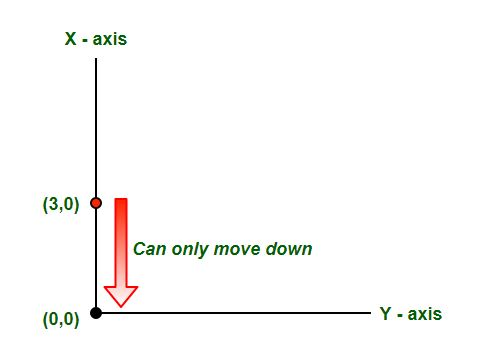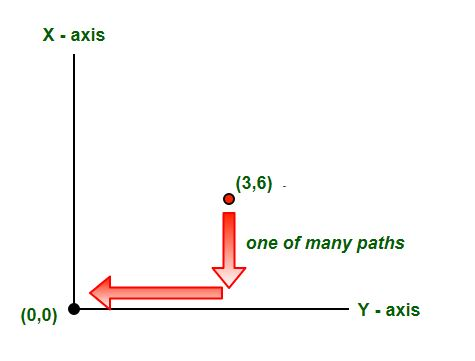Open in App
Not now

# Counts paths from a point to reach Origin

• Difficulty Level : Easy
• Last Updated : 16 Nov, 2022

You are standing on a point (n, m) and you want to go to origin (0, 0) by taking steps either left or down i.e. from each point you are allowed to move either in (n-1, m) or (n, m-1). Find the number of paths from point to origin.

Examples:

```Input : 3 6
Output : Number of Paths 84

Input : 3 0
Output : Number of Paths 1```Recommended Practice

As we are restricted to move down and left only we would run a recursive loop for each of the combinations of the
steps that can be taken.

```// Recursive function to count number of paths
countPaths(n, m)
{
// If we reach bottom or top left, we are
// have only one way to reach (0, 0)
if (n==0 || m==0)
return 1;

// Else count sum of both ways
return (countPaths(n-1, m) + countPaths(n, m-1));
} ```

Below is the implementation of the above steps.

## C++

 `// C++ program to count total number of``// paths from a point to origin``#include``using` `namespace` `std;` `// Recursive function to count number of paths``int` `countPaths(``int` `n, ``int` `m)``{``    ``// If we reach bottom or top left, we are``    ``// have only one way to reach (0, 0)``    ``if` `(n==0 || m==0)``        ``return` `1;` `    ``// Else count sum of both ways``    ``return` `(countPaths(n-1, m) + countPaths(n, m-1));``}` `// Driver Code``int` `main()``{``    ``int` `n = 3, m = 2;``    ``cout << ``" Number of Paths "` `<< countPaths(n, m);``    ``return` `0;``}`

## Java

 `// Java program to count total number of``// paths from a point to origin``import` `java.io.*;` `class` `GFG {``    ` `    ``// Recursive function to count number of paths``    ``static` `int` `countPaths(``int` `n, ``int` `m)``    ``{``        ``// If we reach bottom or top left, we are``        ``// have only one way to reach (0, 0)``        ``if` `(n == ``0` `|| m == ``0``)``            ``return` `1``;``    ` `        ``// Else count sum of both ways``        ``return` `(countPaths(n - ``1``, m) + countPaths(n, m - ``1``));``    ``}``    ` `    ``// Driver Code``    ``public` `static` `void` `main (String[] args)``    ``{``        ``int` `n = ``3``, m = ``2``;``        ``System.out.println (``" Number of Paths "``                            ``+ countPaths(n, m));``        ` `    ``}``}` `// This code is contributed by vt_m`

## Python3

 `# Python3 program to count``# total number of``# paths from a point to origin``# Recursive function to``# count number of paths``def` `countPaths(n,m):` `    ``# If we reach bottom``    ``# or top left, we are``    ``# have only one way to reach (0, 0)``    ``if` `(n``=``=``0` `or` `m``=``=``0``):``        ``return` `1`` ` `    ``# Else count sum of both ways``    ``return` `(countPaths(n``-``1``, m) ``+` `countPaths(n, m``-``1``))` `# Driver Code``n ``=` `3``m ``=` `2``print``(``" Number of Paths "``, countPaths(n, m))` `# This code is contributed``# by Azkia Anam.`

## C#

 `// C# program to count total number of``// paths from a point to origin``using` `System;``        ` `public` `class` `GFG {``    ` `    ``// Recursive function to count number``    ``// of paths``    ``static` `int` `countPaths(``int` `n, ``int` `m)``    ``{``        ` `        ``// If we reach bottom or top left,``        ``// we are have only one way to``        ``// reach (0, 0)``        ``if` `(n == 0 || m == 0)``            ``return` `1;``    ` `        ``// Else count sum of both ways``        ``return` `(countPaths(n - 1, m)``                 ``+ countPaths(n, m - 1));``    ``}``    ` `    ``// Driver Code``    ``public` `static` `void` `Main ()``    ``{``        ``int` `n = 3, m = 2;``        ` `        ``Console.WriteLine (``" Number of"``         ``+ ``" Paths "` `+ countPaths(n, m));``        ` `    ``}``}` `// This code is contributed by Sam007.`

## PHP

 ``

## Javascript

 ``

Output

` Number of Paths 10`

We can use Dynamic Programming as there are overlapping subproblems. We can draw recursion tree to see overlapping problems. For example, in case of countPaths(4, 4), we compute countPaths(3, 3) multiple times.

## C++

 `// C++ program to count total number of``// paths from a point to origin``#include``using` `namespace` `std;` `// DP based function to count number of paths``int` `countPaths(``int` `n, ``int` `m)``{``    ``int` `dp[n+1][m+1];` `    ``// Fill entries in bottommost row and leftmost``    ``// columns``    ``for` `(``int` `i=0; i<=n; i++)``      ``dp[i] = 1;``    ``for` `(``int` `i=0; i<=m; i++)``      ``dp[i] = 1;` `    ``// Fill DP in bottom up manner``    ``for` `(``int` `i=1; i<=n; i++)``       ``for` `(``int` `j=1; j<=m; j++)``          ``dp[i][j] = dp[i-1][j] + dp[i][j-1];` `    ``return` `dp[n][m];``}` `// Driver Code``int` `main()``{``    ``int` `n = 3, m = 2;``    ``cout << ``" Number of Paths "` `<< countPaths(n, m);``    ``return` `0;``}`

## Java

 `// Java program to count total number of``// paths from a point to origin``import` `java.io.*;` `class` `GFG {``    ` `    ``// DP based function to count number of paths``    ``static` `int` `countPaths(``int` `n, ``int` `m)``    ``{``        ``int` `dp[][] = ``new` `int``[n + ``1``][m + ``1``];``    ` `        ``// Fill entries in bottommost row and leftmost``        ``// columns``        ``for` `(``int` `i = ``0``; i <= n; i++)``            ``dp[i][``0``] = ``1``;``        ``for` `(``int` `i = ``0``; i <= m; i++)``            ``dp[``0``][i] = ``1``;``    ` `        ``// Fill DP in bottom up manner``        ``for` `(``int` `i = ``1``; i <= n; i++)``        ``for` `(``int` `j = ``1``; j <= m; j++)``            ``dp[i][j] = dp[i - ``1``][j] + dp[i][j - ``1``];``    ` `        ``return` `dp[n][m];``    ``}``    ` `    ``// Driver Code``    ``public` `static` `void` `main (String[] args) {``        ``int` `n = ``3``, m = ``2``;``        ``System.out.println(``" Number of Paths "``                           ``+ countPaths(n, m));``        ` `    ``}``}` `// This code is contributed by vt_m`

## Python3

 `# Python3 program to count total``# number of paths from a point to origin` `# Recursive function to count``# number of paths``def` `countPaths(n, m):` `    ``# If we reach bottom or top``    ``# left, we are have only one``    ``# way to reach (0, 0)``    ``if` `(n ``=``=` `0` `or` `m ``=``=` `0``):``        ``return` `1` `    ``# Else count sum of both ways``    ``return` `(countPaths(n ``-` `1``, m) ``+``            ``countPaths(n, m ``-` `1``))` `# Driver Code``n ``=` `3``m ``=` `2``print``(``"Number of Paths"``,``       ``countPaths(n, m))` `# This code is contributed by ash264`

## C#

 `// C# program to count total number of``// paths from a point to origin``using` `System;``        ` `public` `class` `GFG {``    ` `    ``// DP based function to count number``    ``// of paths``    ``static` `int` `countPaths(``int` `n, ``int` `m)``    ``{``        ``int` `[,]dp = ``new` `int``[n + 1,m + 1];``    ` `        ``// Fill entries in bottommost row``        ``// and leftmost columns``        ``for` `(``int` `i = 0; i <= n; i++)``            ``dp[i,0] = 1;``        ``for` `(``int` `i = 0; i <= m; i++)``            ``dp[0,i] = 1;``    ` `        ``// Fill DP in bottom up manner``        ``for` `(``int` `i = 1; i <= n; i++)``            ``for` `(``int` `j = 1; j <= m; j++)``                ``dp[i,j] = dp[i - 1,j]``                         ``+ dp[i,j - 1];``        ` `        ``return` `dp[n,m];``    ``}``    ` `    ``// Driver Code``    ``public` `static` `void` `Main ()``    ``{``        ``int` `n = 3, m = 2;``        ` `        ``Console.WriteLine(``" Number of"``        ``+ ``" Paths "` `+ countPaths(n, m));``        ` `    ``}``}` `// This code is contributed by Sam007.`

## PHP

 ``

## Javascript

 ``

Output

` Number of Paths 10`

Time Complexity: O(n*m)
Auxiliary Space: O(n*m)

Another Approach:

Using Pascal’s Triangle Approach, we also solve the problem by calculating the value of n+mCn. It can be observed as a pattern when you increase the value of m keeping the value of n constant.
Below is the implementation of the above approach:

Implementation:

## C++

 `// C++ Program for above approach``#include ``#include ``using` `namespace` `std;` `// Function to find``// binomial Coefficient``int` `binomialCoeff(``int` `n, ``int` `k)``{``    ``int` `C[k+1];``    ``memset``(C, 0, ``sizeof``(C));``    ``C = 1;``  ` `    ``// Constructing Pascal's Triangle``    ``for` `(``int` `i = 1; i <= n; i++)``    ``{``        ``for` `(``int` `j = min(i, k); j > 0; j--)``            ``C[j] = C[j] + C[j-1];``    ``}``    ``return` `C[k];``}` `//Driver Code``int` `main()``{``    ``int` `n=3, m=2;``    ``cout<<``"Number of Paths: "``<<``                ``binomialCoeff(n+m,n)<

## Java

 `// Java Program for above approach``import` `java.io.*;``import` `java.util.*;` `class` `GFG``{``    ``static` `int` `min(``int` `a,``int` `b)``    ``{``        ``return` `a ``0``; j--)``                ``C[j] = C[j] + C[j-``1``];``        ``}``        ``return` `C[k];``    ``}``  ` `    ``// Driver Code``    ``public` `static` `void` `main (String[] args)``    ``{``        ``int` `n=``3``,m=``2``;``        ``System.out.println(``"Number of Paths: "` `+``                           ``binomialCoeff(n+m,n));``    ``}``}``//Contributed by Vismay_7`

## Python3

 `# Python3 program for above approach``def` `binomialCoeff(n,k):``    ` `    ``C ``=` `[``0``]``*``(k``+``1``)``    ``C[``0``] ``=` `1``    ` `    ``# Computing Pascal's Triangle``    ``for` `i ``in` `range``(``1``, n ``+` `1``):``        ` `        ``j ``=` `min``(i ,k)``        ``while` `(j > ``0``):``            ``C[j] ``=` `C[j] ``+` `C[j``-``1``]``            ``j ``-``=` `1``  ` `    ``return` `C[k]``  ` `# Driver Code``n``=``3``m``=``2``print``(``"Number of Paths:"``,binomialCoeff(n``+``m,n))` `# Contributed by Vismay_7`

## C#

 `// C# program for above approach``using` `System;` `class` `GFG{``    ` `// Function to find``// binomial Coefficient``static` `int` `binomialCoeff(``int` `n, ``int` `k)``{``    ``int``[] C = ``new` `int``[k + 1];``    ``C = 1;``   ` `    ``// Constructing Pascal's Triangle``    ``for``(``int` `i = 1; i <= n; i++)``    ``{``        ``for``(``int` `j = Math.Min(i, k); j > 0; j--)``            ``C[j] = C[j] + C[j - 1];``    ``}``    ``return` `C[k];``}` `// Driver code``static` `void` `Main()``{``    ``int` `n = 3, m = 2;``    ` `    ``Console.WriteLine(``"Number of Paths: "` `+``                      ``binomialCoeff(n + m, n));``}``}` `// This code is contributed by divyesh072019`

## Javascript

 ``

Output

`Number of Paths: 10`

Time Complexity : O((m+n)*n)
Auxiliary Space : O(n)

This article is contributed by Nikhil Rawat.If you like GeeksforGeeks and would like to contribute, you can also write an article using write.geeksforgeeks.org or mail your article to review-team@geeksforgeeks.org. See your article appearing on the GeeksforGeeks main page and help other Geeks.

My Personal Notes arrow_drop_up